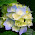## Pages

The greatest mistake you can make in life is to be continually fearing you will make one

## Monday, 8 February 2010

### Infix To Postfix Conversion

Introduction:
In the last post I have explained about the infix,prefix,postfix expression. In this post we are going to know about the conversion of infix to postfix expression.

Infix to Postfix Conversion:
• Consider an infix string x+y*c.Each character of the string is called tokens.
• The algorithm for converting an infix expression to postfix expression is given below.
• We need a stack to solve this problem
Algorithm for converting infix expression to postfix expression:
• Initialize an empty stack and a Postfix String S.
• Read the tokens from the infix string one at a time from left to right
• If the token is an operand, add it to the Postfix string S.
• If the token is a left parentheses, Push it on to the stack
• If the token is a right parentheses
1. Repeatedly Pop the stack and add the poped out element in to the string S until a left parenthesis is encountered;
2. Remove the left parenthesis from the stack and ignore it
• If the token is an operator
1. If the stack is empty push the operator in to the stack
2. If the stack is not empty compare the precedence of the operator with the element on top of the stack
• If the operator in the stack has higher precedence than the token Pop the stack and add the poped out element in to the string S. else Push the operator in to the stack
• Repeat this step until the stack is not empty or the operator in the stack has higher precedence than the token
• Repeat this step till all the characters are read.
• After all the characters are read and the stack is not empty then Pop the stack and add the tokens to the string S
• Return the Postfix string S
Example for Converting infix to Postfix Expression:

Example 1:
Infix String----->A*B+C/D
Solution:
1. Initially the stack is empty and the Postfix String S has no characters
2. Read the token A.A is a operand so it is added to the string S.now Stack->empty and S->A
3. Read the token *.* is an operator.stack is empty so push * in to the stack. now Stack->* and S->A
4. Read the token B.B is an operand so it is added to the string S. now Stack->* and S->AB
5. Read the token +.+is an operator.compare +and *.* has higher precedence than +. so pop * from the stack and add it to the string S. now Stack->empty and S->AB*.now the stack is empty so push the + in to the stack.now Stack->+ and S->AB*
6. Read the token C.C is a operand so it is added to the string S.now Stack->+ and S->AB*C
7. Read the token /./ is an operator.compare /and +./ has higher precedence than +. so push / in to the stack . now Stack->+/ and S->AB*C
8. Read the token D.D is a operand so it is added to the string S.now Stack->+/ and S->AB*CD
9. All the characters are read but the stack is not empty so pop the stack and add the characters to the String. now S->AB*CD/+
So the Postfix String is AB*CD/+

Example 2:
Infix String----->A*(B+C)/D
Solution:
1. Initially the stack is empty and the Postfix String S has no character.
2. Read the token A.A is a operand so it is added to the string S.now Stack->empty and S->A
3. Read the token *.* is an operator.stack is empty so push * in to the stack. now Stack->* and S->A
4. Read the token (.push the left parentheses in to the stack.
5. Read the token B.B is an operand so it is added to the string S. now Stack->* and S->AB
6. Read the token +.+is an operator.compare +and *.+ has lower precedence than *.So push + in to the stack. now Stack->*+ and S->AB
7. Read the token C.C is an operand so it is added to the string S.now Stack->*+ and S->ABC
8. Read the token ). ) is a right parentheses ,Pop the stack and add the + in to the string S. now Stack->* and S->ABC+
9. Read the token /./ is an operator.compare /and *./ and * have equal precedence but * comes before /so pop the stack and add * in to the string S.now stack is empty so push / in to the stack . now Stack->/ and S->ABC+*
10. Read the token D.D is an operand so it is added to the string S.now Stack->/ and S->ABC+*D
11. Now the input string is empty but the stack is not empty so pop the stack and add the characters to the String.now Stack->Empty and S->ABC+*D/
So the Postfix String is ABC+*D/

Exercise for Converting infix to Postfix Expression:
1. A+B-C
2. A+B*C
3. (A+B)*C
4. (A+B)*(C-D)
5. ((A+B)*C-(D-E))%(F+G)
6. A*(B+C/D)
7. ((A*B)+(C/D))
8. ((A*(B+C))/D)
9. (A*(B+(C/D)))
10. (2+((3+4)*(5*6)))

1.hey thnx,now i can convert infix to postfix,thnx for ur help.

2.3.extremely helfpful

1.2.4.5.very understandable.....thanx....

6.thanx for such a easy type code

7.no use......

8.ok fine

9.This is BULLSHIT! I NEED A PROGRAM! WATDAFUCK!

1.This comment has been removed by the author.

2.10.You have explained it so beautifully.
Thank yo so much :)

11.excelent tutorial .. i am bookmarking it :)

12.very gud

13.This is fine... What to do in the case of unary operators....
for example, infix statement: -a+b
what is its postfix statement?????

14.15.what if the given output should be in step by step solution??? can you help me guyz?

16.C code of infix to postfix http://thecprojectt.blogspot.in/2013/03/c-program-15_27.html

17.thanks.... it is easy and understandable

18.thanks.. it helps..